Next: Projectile Motion with Air Up: Multi-Dimensional Motion Previous: Introduction

# Motion in a Two-Dimensional Harmonic Potential

Consider a particle of massmoving in the two-dimensional harmonic potential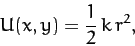(154)

where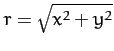, and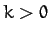. It follows that the particle is subject to a force,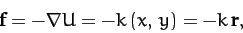(155)

which always points towards the origin, and whose magnitude increases linearly with increasing distance from the origin. According to Newton's second law, the equation of motion of the particle is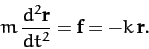(156)

When written in component form, the above equation reduces to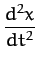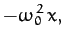(157)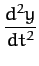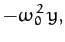(158)

where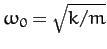.

Since Equations (157) and (158) are both simple harmonic equations, we can immediately write their general solutions: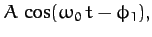(159)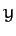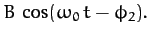(160)

Here,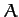,,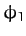, and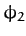are arbitrary constants of integration. We can simplify the above equations slightly by shifting the origin of time (which is, after all, arbitrary): i.e.,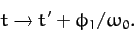(161)

Hence, we obtain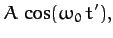(162)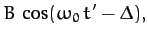(163)

where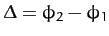. Note that the motion is clearly periodic in time, with period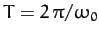. Thus, the particle must trace out some closed trajectory in the-plane. The question, now, is what does this trajectory look like as a function of the relative phase-shift,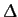, between the oscillations in the- and-directions?

Using standard trigonometry, we can write Equation (163) in the form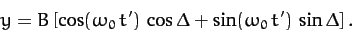(164)

Hence, using Equation (162), we obtain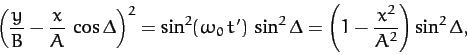(165)

which simplifies to give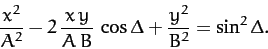(166)

Unfortunately, the above equation is not immediately recognizable as being the equation of any particular geometric curve: e.g., a circle, an ellipse, or a parabola, etc.

Perhaps our problem is that we are using the wrong coordinates. Suppose that we rotate our coordinate axes about the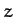-axis by an angle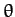, as illustrated in Figure A.100. According to Equations (A.1277) and (A.1278), our old coordinates (,) are related to our new coordinates (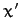,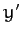) via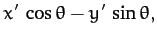(167)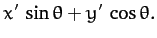(168)

Let us see whether Equation (166) takes a simpler form when expressed in terms of our new coordinates. Equations (166)-(168) yield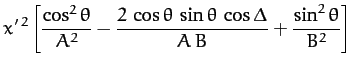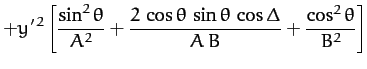(169)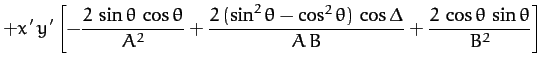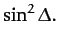We can simplify the above equation by setting the term involving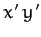to zero. Hence,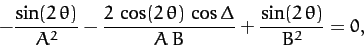(170)

where we have made use of some simple trigonometric identities. Thus, theterm disappears whentakes the special value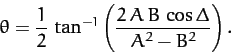(171)

In this case, Equation (169) reduces to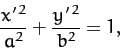(172)

where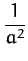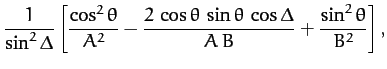(173)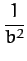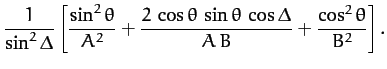(174)

Of course, we immediately recognize Equation (172) as the equation of an ellipse, centered on the origin, whose major and minor axes are aligned along the- and-axes, and whose major and minor radii are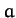and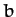, respectively (assuming that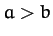).

We conclude that, in general, a particle of massmoving in the two-dimensional harmonic potential (154) executes a closed elliptical orbit (which is not necessarily aligned along the- and-axes), centered on the origin, with period, where.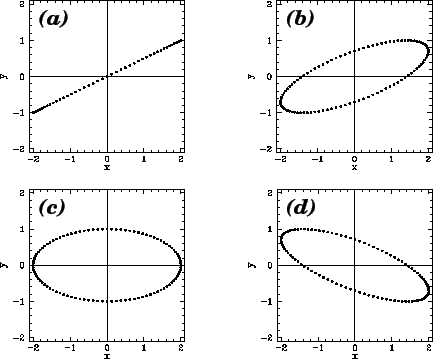Figure 10 shows some example trajectories calculated for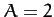,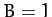, and the following values of the phase difference,: (a)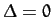; (b)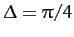; (c)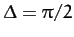; (d)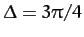. Note that whenthe trajectory degenerates into a straight-line (which can be thought of as an ellipse whose minor radius is zero).

Perhaps, the main lesson to be learned from the above study of two-dimensional motion in a harmonic potential is that comparatively simple patterns of motion can be made to look complicated when expressed in terms of ill-chosen coordinates.Next: Projectile Motion with Air Up: Multi-Dimensional Motion Previous: Introduction
Richard Fitzpatrick 2011-03-31